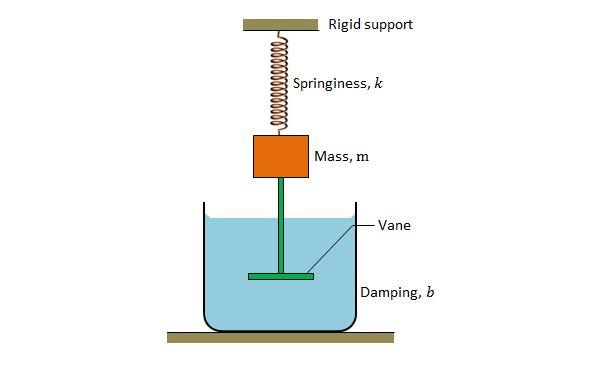Classical Mechanics

# Damping and amplitude decreasesThe above is a schematic diagram of a damped oscillator, where the block has a mass of $$m=8.0\text{ kg},$$ spring constant $$k=130\text{ N/m},$$ and damping constant $$b=70\text{ g/s}.$$ Approximately, how long does it take for the mechanical energy to drop to one-half its initial value?The above is a schematic diagram of a damped oscillator, where the block has a mass of $$1.7\text{ kg}$$ and the spring constant is $$9\text{ N/m}.$$ The damping force can be expressed as $$-b(dx/dt),$$ where $$b=210\text{ g/s}.$$ If the block is pulled down $$12.0\text{ cm}$$ and released, approximately, what is the time required for the amplitude of the resulting oscillations to fall to one-third of its initial value?The above is a schematic diagram of a damped oscillator. If the damped oscillator has mass $$m=240\text{ g},$$ spring constant $$k=85\text{ N/m},$$ and damping constant $$b=70\text{ g/s},$$ what is the approximate ratio of the amplitude of the damped oscillations to the initial amplitude at the end of $$10$$ cycles?The above is a schematic diagram of a damped oscillator, where the block has a mass of $$m=250\text{ g}$$ and the spring constant is $$k=95 \text{ N/m}.$$ It takes $$4.0\text{ s}$$ for the amplitude of the damped oscillations to drop to half its initial value. If we replace the block with mass $$4m$$ and the spring with spring constant $$3k,$$ how long will it take for the amplitude of the damped oscillations to drop to half its initial value?The above is a schematic diagram of a damped oscillator, where the block has a mass of $$m$$ and spring constant $$k.$$ If the ratio of the amplitude of the damped oscillations at the end of $$30$$ cycles to the initial amplitude is $$0.57,$$ what will be the ratio of the amplitude of the damped oscillations at the end of $$60$$ cycles to the initial amplitude?

×

Problem Loading...

Note Loading...

Set Loading...